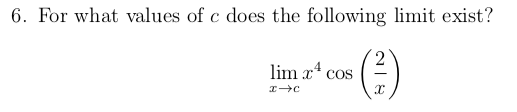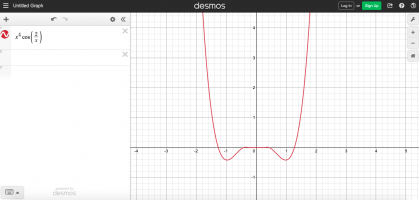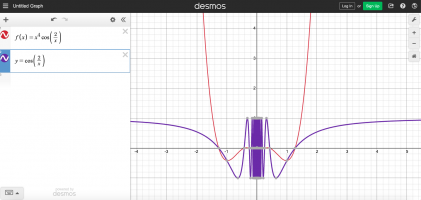Finding the Values of "c" for a Limit

kmunzenmaier

New member
Hi everyone!

I'm really stuck on this question and can't seem to find any related examples online to help me with understanding how to solve the problem (I'm having a hard time with the Midterm Review for the Calculus class I'm currently enrolled in and will probably be getting a math tutor this week or the next); is there an example of a similar type of question to use for applying to the problem I'm stuck on? Here's a screenshot of the question:I'm not sure how to find the value of c from the given function (I've never encountered this problem in the homework or quizzes for the class); it appears that the domain is all real numbers except for 0 where the given function would be undefined, but I haven't made much progress with this question as far as the domain is concerned :/

I want to do really well on the Calculus Midterm and would love to receive any feedback for this question if possible ~ thank you!Subhotosh Khan

Super Moderator
Staff member
Hi everyone!

I'm really stuck on this question and can't seem to find any related examples online to help me with understanding how to solve the problem (I'm having a hard time with the Midterm Review for the Calculus class I'm currently enrolled in and will probably be getting a math tutor this week or the next); is there an example of a similar type of question to use for applying to the problem I'm stuck on? Here's a screenshot of the question:

View attachment 29199
I'm not sure how to find the value of c from the given function (I've never encountered this problem in the homework or quizzes for the class); it appears that the domain is all real numbers except for 0 where the given function would be undefined, but I haven't made much progress with this question as far as the domain is concerned :/

I want to do really well on the Calculus Midterm and would love to receive any feedback for this question if possible ~ thank you!If I were to do this problem, I would first plot y = cos(2/x) and study the plot. Then look at y = x4 * cos(2/x).

Please tell us what you found.

Dr.Peterson

Elite Member
I'm not sure how to find the value of c from the given function (I've never encountered this problem in the homework or quizzes for the class); it appears that the domain is all real numbers except for 0 where the given function would be undefined, but I haven't made much progress with this question as far as the domain is concerned :/
Having looked at the domain, you should also decide whether the function is continuous everywhere in its domain. If it is, then you only need to consider whether it has a limit at x=0. (Do you see why?)

What methods have you learned for finding such limits?

kmunzenmaier

New member
If I were to do this problem, I would first plot y = cos(2/x) and study the plot. Then look at y = x4 * cos(2/x).

Please tell us what you found.

Hi!

Thank you for the tip; I used Desmos to get an idea of where this function has a limit and it appears that it's continuous everywhere except for at x=0 where it's undefined (I think that there's a limit for every value of "c" based on the graph though; I don't see any regions on the function where the left-hand limit doesn't equal the right-hand limit and I think the limit can still exist for areas where there are "holes" on the graph of the function)However, when I graphed the function y=cos(2/x), it appears that the limit doesn't exist for the value x=0 due to the oscillating waves getting closer to this value of "c" which makes it difficult to determine a specific value for the limit.Would it be correct to say that for the original given function, the values of "c" that exist are "all real values of c"?

Last edited:

kmunzenmaier

New member
Having looked at the domain, you should also decide whether the function is continuous everywhere in its domain. If it is, then you only need to consider whether it has a limit at x=0. (Do you see why?)

What methods have you learned for finding such limits?
Hi!

Thank you for the feedback ~ I looked at the graph of the given function on Desmos and it appears that the function is continuous everywhere in its domain except for at x=0 (f(0) where x=0 is undefined which violates the first rule of continuity from what I've learned) Do we only need to consider if the function has a limit at x=0 when the functon is continuous to ensure that it's not an oscillating function with no limit at that central point? (I'm not sure)

I've never been given this type of question before in the homework or quizzes so I'm not sure which methods I should use to tackle the problem (I definitely wouldn't be allowed to use Desmos during the exam so I'd probably have to tackle this question algebraically) I know that l'Hopital's Rule is used for the more difficult calculation of limits but I'm not sure if it applies to this specific problem; I'll have to ask my instructor if an answer sheet will be given soon for the Midterm Review so I can check my answersI'm leaning toward answering as "all real values of c" for this question but I'm still not sure :/ Thank you for the help!Dr.Peterson

Elite Member
Hi!

Thank you for the feedback ~ I looked at the graph of the given function on Desmos and it appears that the function is continuous everywhere in its domain except for at x=0 (f(0) where x=0 is undefined which violates the first rule of continuity from what I've learned) Do we only need to consider if the function has a limit at x=0 when the functon is continuous to ensure that it's not an oscillating function with no limit at that central point? (I'm not sure)

I've never been given this type of question before in the homework or quizzes so I'm not sure which methods I should use to tackle the problem (I definitely wouldn't be allowed to use Desmos during the exam so I'd probably have to tackle this question algebraically) I know that l'Hopital's Rule is used for the more difficult calculation of limits but I'm not sure if it applies to this specific problem; I'll have to ask my instructor if an answer sheet will be given soon for the Midterm Review so I can check my answersI'm leaning toward answering as "all real values of c" for this question but I'm still not sure :/ Thank you for the help!L'Hopital doesn't apply here; but the cosine is bounded and the other factor approaches zero. This justifies what you see in the graph, that the product approaches zero. You may have learned a theorem to that effect.

So, yes, the limit exists for all c, including zero.

•lev888